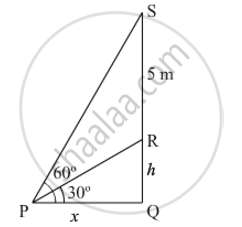# From a point P on the ground the angle of elevation of the top of a tower is 30° and that of the top of a flag staff fixed on the top of the tower, is 60°. If the length of the flag staff is 5 m, find the height of the tower. - Mathematics

From a point P on the ground the angle of elevation of the top of a tower is 30° and that of the top of a flag staff fixed on the top of the tower, is 60°. If the length of the flag staff is 5 m, find the height of the tower.

#### SolutionLet RQ be the tower and SR be the flag staff.

In PQR,

tan30^@=(RQ)/(PQ)

=>1/sqrt3=h/x

=>x=hsqrt3 " .....(i)"

In PQS

tan 60^@=(SQ)/(PQ)

=>sqrt3=(h+5)/x

=>xsqrt()3=h+5" .....(ii)"

From (i) and (ii), we get

3h=h+5

2h=5

h=2.5 m

Hence, the height of the tower is 2.5 metres.

Concept: Heights and Distances
Is there an error in this question or solution?